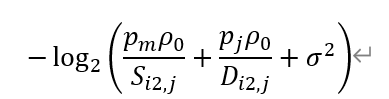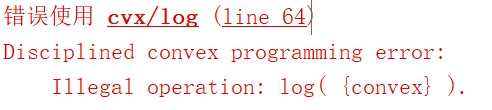# CVX solves convex optimization problems

When I run the following formula：Where p_m,p_j, ρ_0, σ^2 are constant,S and H are the relaxation variables.I encountered the following error：.How can I solve it

Section 5,2.7 of the Mosek Modeling Cookbook https://docs.mosek.com/modeling-cookbook/expo.html#modeling-with-the-exponential-cone shows two ways to do it, the first of which makes use of CVX’s `log_sum_exp`

If you study the whole cookbook, it should improve your ability to use CVX.